# 12. Output devices¶

## Group Assignment : measure the power consumption of an output device¶

For an output device, we chose LED. The below is the design of the board with LED , two types of resistance(1kΩ and 100Ω), and shunt resistance.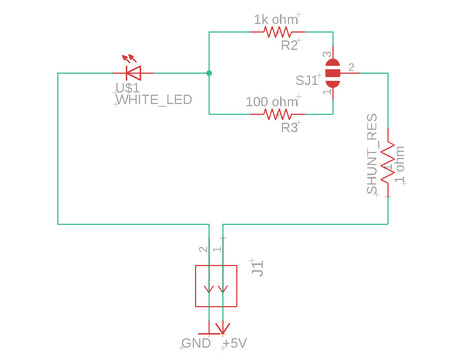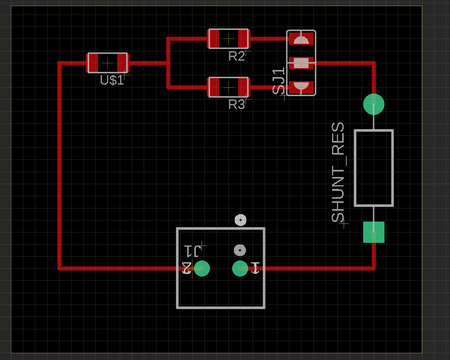To figure out the color code of a shunt resistance, there is a useful app of Degikey Color Code Calcurator.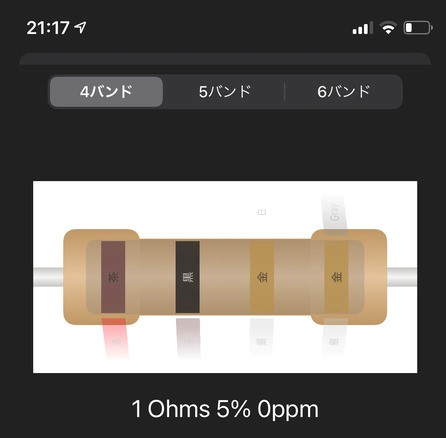In this board, we could switch easily the resistor to use by changing the position of a jumper pin on the upper right.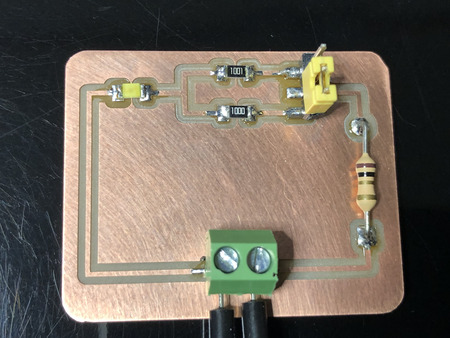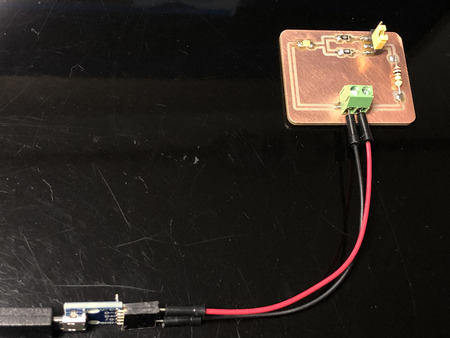Before measurement, we needed to understand the basic formula of the electricity.

• Ohm’s law V(Voltage) = I (Intensity of electricity)x R(Resistance)

• P = I x V [W]

The multimeter was necessary to measure voltage.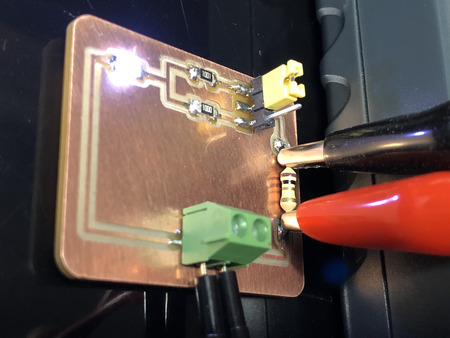### ■In the case of 1kΩ¶

#### 1.Measure voltage of each part.¶

A.Supply voltage:5.08V

B.Shunt resistance of 1Ω:2.4mV

C.Both ends of 1kΩ:2.36V

D.Both ends of LED:2.71V

A=B+C+D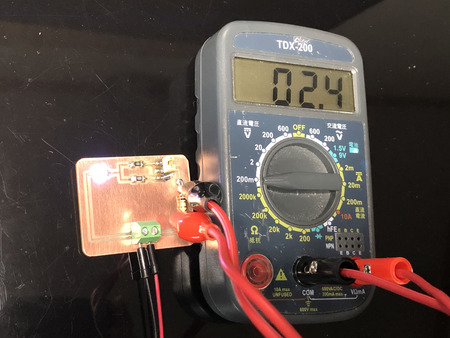#### 2.Calcurate intensity of electricity by a shunt resistance.¶

V=IR (Ohm’s low)

I=V/R=2.4mV/1Ω=2.4mA(intensity of electricity in a shunt resistance.)

There should be same amount of electricity in LED and 1kΩ resistor.

#### 3.Calculate voltage of 1kΩ resistor¶

V=IR V=2.4mA×1kΩ=2.4X1000mV=2400mV=2.4V

#### 4.Calculate the power consumption of an output device(LED)¶

P[W]=IV=2.4mA×2.71V=6.504mW

#### 5.Calculate the power consumption of whole circuit¶

P[W]=IV=2.4mA×5.08V=12.192mW

### ■In the case of 100Ω¶

#### 1.Measure voltage of each part.¶

A.Supply voltage:5.07V

B.Shunt resistance of 1Ω:19.2mV

C.Both ends of 100Ω:1.95V

D.Both ends of LED:3.10V

A=B+C+D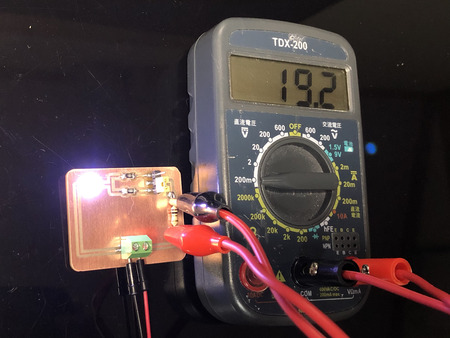#### 2.Calcurate intensity of electricity by a shunt resistance.¶

V=IR (Ohm’s low)

I=V/R=19.2mV/1Ω=19,2mA(intensity of electricity in a shunt resistance.)

There should be same amount of electricity in LED and 100Ω resistor.

#### 3.Calculate voltage of 1kΩ resistor¶

V=IR

V=19.2mA×100Ω=19.2X100mV=1920mV=1.92V

#### 4.Calculate the power consumption of an output device(LED)¶

P[W]=IV=19.2mA×1.92V=36.864mW

#### 5.Calculate the power consumption of whole circuit¶

P[W]=IV=19,2mA×5.07V=97.344mW

Last update: June 17, 2021